# `sklearn.pipeline`.Pipeline¶

class `sklearn.pipeline.``Pipeline`(steps, memory=None)[source]

Pipeline of transforms with a final estimator.

Sequentially apply a list of transforms and a final estimator. Intermediate steps of the pipeline must be ‘transforms’, that is, they must implement fit and transform methods. The final estimator only needs to implement fit. The transformers in the pipeline can be cached using `memory` argument.

The purpose of the pipeline is to assemble several steps that can be cross-validated together while setting different parameters. For this, it enables setting parameters of the various steps using their names and the parameter name separated by a ‘__’, as in the example below. A step’s estimator may be replaced entirely by setting the parameter with its name to another estimator, or a transformer removed by setting to None.

Read more in the User Guide.

Parameters: steps : list List of (name, transform) tuples (implementing fit/transform) that are chained, in the order in which they are chained, with the last object an estimator. memory : None, str or object with the joblib.Memory interface, optional Used to cache the fitted transformers of the pipeline. By default, no caching is performed. If a string is given, it is the path to the caching directory. Enabling caching triggers a clone of the transformers before fitting. Therefore, the transformer instance given to the pipeline cannot be inspected directly. Use the attribute `named_steps` or `steps` to inspect estimators within the pipeline. Caching the transformers is advantageous when fitting is time consuming. named_steps : bunch object, a dictionary with attribute access Read-only attribute to access any step parameter by user given name. Keys are step names and values are steps parameters.

Examples

```>>> from sklearn import svm
>>> from sklearn.datasets import samples_generator
>>> from sklearn.feature_selection import SelectKBest
>>> from sklearn.feature_selection import f_regression
>>> from sklearn.pipeline import Pipeline
>>> # generate some data to play with
>>> X, y = samples_generator.make_classification(
...     n_informative=5, n_redundant=0, random_state=42)
>>> # ANOVA SVM-C
>>> anova_filter = SelectKBest(f_regression, k=5)
>>> clf = svm.SVC(kernel='linear')
>>> anova_svm = Pipeline([('anova', anova_filter), ('svc', clf)])
>>> # You can set the parameters using the names issued
>>> # For instance, fit using a k of 10 in the SelectKBest
>>> # and a parameter 'C' of the svm
>>> anova_svm.set_params(anova__k=10, svc__C=.1).fit(X, y)
...
Pipeline(memory=None,
steps=[('anova', SelectKBest(...)),
('svc', SVC(...))])
>>> prediction = anova_svm.predict(X)
>>> anova_svm.score(X, y)
0.829...
>>> # getting the selected features chosen by anova_filter
>>> anova_svm.named_steps['anova'].get_support()
...
array([False, False,  True,  True, False, False, True,  True, False,
True,  False,  True,  True, False, True,  False, True, True,
False, False], dtype=bool)
>>> # Another way to get selected features chosen by anova_filter
>>> anova_svm.named_steps.anova.get_support()
...
array([False, False,  True,  True, False, False, True,  True, False,
True,  False,  True,  True, False, True,  False, True, True,
False, False], dtype=bool)
```

Methods

 `decision_function`(X) Apply transforms, and decision_function of the final estimator `fit`(X[, y]) Fit the model `fit_predict`(X[, y]) Applies fit_predict of last step in pipeline after transforms. `fit_transform`(X[, y]) Fit the model and transform with the final estimator `get_params`([deep]) Get parameters for this estimator. `predict`(X) Apply transforms to the data, and predict with the final estimator `predict_log_proba`(X) Apply transforms, and predict_log_proba of the final estimator `predict_proba`(X) Apply transforms, and predict_proba of the final estimator `score`(X[, y, sample_weight]) Apply transforms, and score with the final estimator `set_params`(**kwargs) Set the parameters of this estimator.
`__init__`(steps, memory=None)[source]
`decision_function`(X)[source]

Apply transforms, and decision_function of the final estimator

Parameters: X : iterable Data to predict on. Must fulfill input requirements of first step of the pipeline. y_score : array-like, shape = [n_samples, n_classes]
`fit`(X, y=None, **fit_params)[source]

Fit the model

Fit all the transforms one after the other and transform the data, then fit the transformed data using the final estimator.

Parameters: X : iterable Training data. Must fulfill input requirements of first step of the pipeline. y : iterable, default=None Training targets. Must fulfill label requirements for all steps of the pipeline. **fit_params : dict of string -> object Parameters passed to the `fit` method of each step, where each parameter name is prefixed such that parameter `p` for step `s` has key `s__p`. self : Pipeline This estimator
`fit_predict`(X, y=None, **fit_params)[source]

Applies fit_predict of last step in pipeline after transforms.

Applies fit_transforms of a pipeline to the data, followed by the fit_predict method of the final estimator in the pipeline. Valid only if the final estimator implements fit_predict.

Parameters: X : iterable Training data. Must fulfill input requirements of first step of the pipeline. y : iterable, default=None Training targets. Must fulfill label requirements for all steps of the pipeline. **fit_params : dict of string -> object Parameters passed to the `fit` method of each step, where each parameter name is prefixed such that parameter `p` for step `s` has key `s__p`. y_pred : array-like
`fit_transform`(X, y=None, **fit_params)[source]

Fit the model and transform with the final estimator

Fits all the transforms one after the other and transforms the data, then uses fit_transform on transformed data with the final estimator.

Parameters: X : iterable Training data. Must fulfill input requirements of first step of the pipeline. y : iterable, default=None Training targets. Must fulfill label requirements for all steps of the pipeline. **fit_params : dict of string -> object Parameters passed to the `fit` method of each step, where each parameter name is prefixed such that parameter `p` for step `s` has key `s__p`. Xt : array-like, shape = [n_samples, n_transformed_features] Transformed samples
`get_params`(deep=True)[source]

Get parameters for this estimator.

Parameters: deep : boolean, optional If True, will return the parameters for this estimator and contained subobjects that are estimators. params : mapping of string to any Parameter names mapped to their values.
`inverse_transform`

Apply inverse transformations in reverse order

All estimators in the pipeline must support `inverse_transform`.

Parameters: Xt : array-like, shape = [n_samples, n_transformed_features] Data samples, where `n_samples` is the number of samples and `n_features` is the number of features. Must fulfill input requirements of last step of pipeline’s `inverse_transform` method. Xt : array-like, shape = [n_samples, n_features]
`predict`(X)[source]

Apply transforms to the data, and predict with the final estimator

Parameters: X : iterable Data to predict on. Must fulfill input requirements of first step of the pipeline. y_pred : array-like
`predict_log_proba`(X)[source]

Apply transforms, and predict_log_proba of the final estimator

Parameters: X : iterable Data to predict on. Must fulfill input requirements of first step of the pipeline. y_score : array-like, shape = [n_samples, n_classes]
`predict_proba`(X)[source]

Apply transforms, and predict_proba of the final estimator

Parameters: X : iterable Data to predict on. Must fulfill input requirements of first step of the pipeline. y_proba : array-like, shape = [n_samples, n_classes]
`score`(X, y=None, sample_weight=None)[source]

Apply transforms, and score with the final estimator

Parameters: X : iterable Data to predict on. Must fulfill input requirements of first step of the pipeline. y : iterable, default=None Targets used for scoring. Must fulfill label requirements for all steps of the pipeline. sample_weight : array-like, default=None If not None, this argument is passed as `sample_weight` keyword argument to the `score` method of the final estimator. score : float
`set_params`(**kwargs)[source]

Set the parameters of this estimator.

Valid parameter keys can be listed with `get_params()`.

Returns: self :
`transform`

Apply transforms, and transform with the final estimator

This also works where final estimator is `None`: all prior transformations are applied.

Parameters: X : iterable Data to transform. Must fulfill input requirements of first step of the pipeline. Xt : array-like, shape = [n_samples, n_transformed_features]

## Examples using `sklearn.pipeline.Pipeline`¶Feature Union with Heterogeneous Data Sources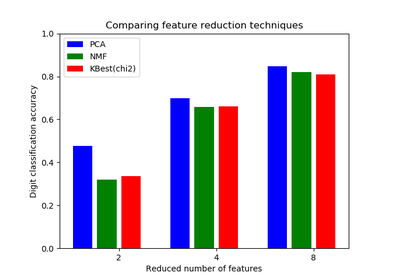Selecting dimensionality reduction with Pipeline and GridSearchCV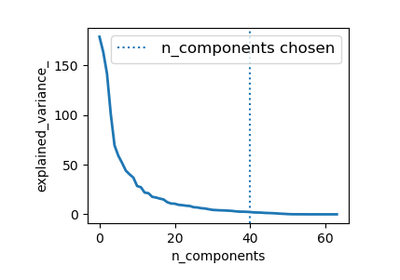Pipelining: chaining a PCA and a logistic regressionConcatenating multiple feature extraction methods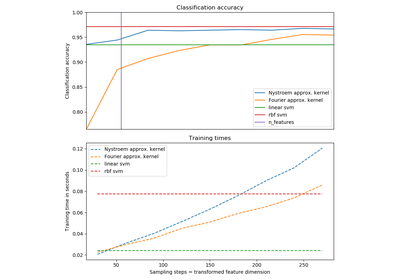Explicit feature map approximation for RBF kernelsImputing missing values before building an estimator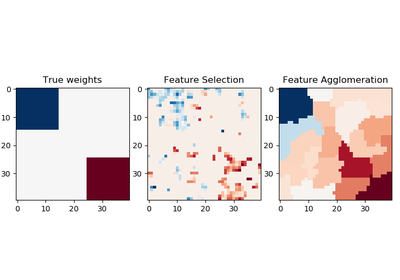Feature agglomeration vs. univariate selectionSample pipeline for text feature extraction and evaluation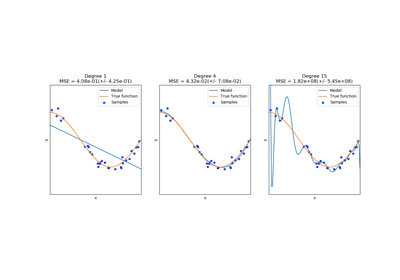Underfitting vs. Overfitting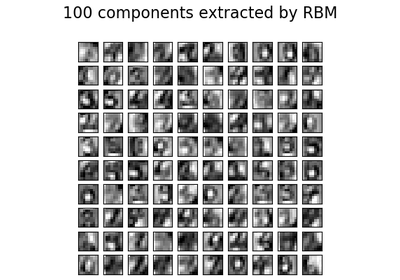Restricted Boltzmann Machine features for digit classification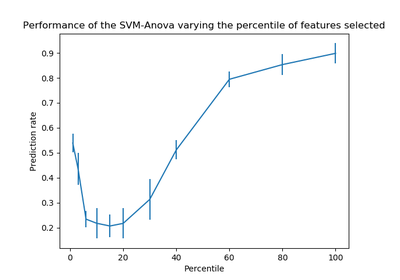SVM-Anova: SVM with univariate feature selectionClassification of text documents using sparse features